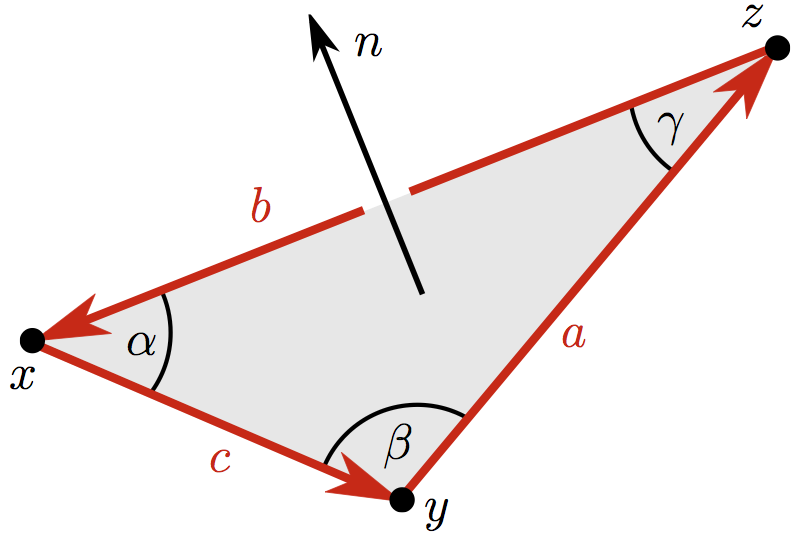# Clayton ShonkwilerIn our paper “Generalized Gauss maps and integrals for three-component links: Toward higher helicities for magnetic fields and fluid flows, part II”, Dennis DeTurck, Herman Gluck, Rafal Komendarczyk, Paul Melvin, Haggai Nuchi, David Shea Vela-Vick and myself defined a generalization of the Gauss map which detects all link-homotopy invariants of three-component links in $$\mathbb{R}^3$$.

The usual Gauss map is defined as follows: if $$X = \{x(s): s \in S^1\}$$ and $$Y=\{y(t):t \in S^1\}$$ form a two-component link, then the Gauss map is the map $$g: S^1 \times S^1 \to S^2$$ given by $$g(s,t) = \frac{x(s)-y(t)}{\|x(s)-y(t)\|}$$. The degree of the Gauss map is equal to the linking number of the link, and computing that degree explicitly yields the famous Gauss linking integral:

$$Lk(X,Y) = \frac{1}{4\pi} \int_{S^1 \times S^1} \frac{dx}{ds} \times \frac{dy}{dt} \cdot \frac{x(s) - y(t)}{\|x(s)-y(t)\|^3} ds dt$$

In turn, this integral is the key to many physical applications, especially the helicity of a plasma or fluid flow.

Our generalized Gauss map applies to three-component links. Given parametrized components $$X = \{x(s): s \in S^1\}$$, $$Y=\{y(t):t \in S^1\}$$, and $$Z = \{z(u): u \in S^1\}$$, a triple of points $$x(s), y(t),z(u)$$, one from each component, forms a (possibly degenerate) triangle, as in the image above. Letting $$n$$ be the unit normal to this triangle, we define the generalized Gauss map $$G: S^1 \times S^1 \times S^1 \to S^2$$ to be the normalization of

$$\frac{a}{|a|} + \frac{b}{|b|} + \frac{c}{|c|} + (\sin \alpha + \sin \beta + \sin \gamma)n$$

We show that the homotopy invariants of this map are precisely the link-homotopy invariants of the three-component link. In particular, the degrees of its restrictions to the faces of the torus recover the pairwise linking numbers (so it really is a generalization of the Gauss map), and its Hopf invariant gives Milnor’s triple linking number of the link. In turn, this yields a Gauss-type integral formula for the triple linking number.